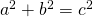# How to use \$ in Articles using WP QuickLaTeX

I use WP QuickLaTeX by Pavel Holoborodko in some of my articles to include mathematical formulas.
Now it can be an issue that the „\$“-sign, that marks the beginning of a formula, is used as dollar sign.

This can be achieved by using [latexpage] in the beginning of the page. Sometimes it is desirable, to apply this only to part of the page. This is achieved using [latexregion].

The following
``` \$ this is a dollar sign [latexregion] And this is a full line formular \$\$a^2+b^2=c^2\$\$ And this is an inline formula \$a^2+b^2=c^2\$. With more stuff... [/latexregion] And here dollar signs \$\$\$\$ are dollar signs again. ```
results in:

\$ this is a dollar sign

And this is a full line formularAnd this is an inline formula. With more stuff…

And here dollar signs \$\$\$\$ are dollar signs again.

And yes, to show the
``` [latexregion] ... [/latexregion] ```
above, I had to actually type
``` &#91;latexregion&#93; ... &#91;/latexregion&#93; ```
Let’s stop the recursion here…